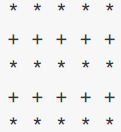# Alternate asterisks square

Hi there, i’m trying to do a pretty simple exercise but I don’t know how to solve the problem; the text exercise is:
Write a program that reads from standard input an integer number n and that, as shown in the Execution example, prints to video a square of n lines consisting each of n symbols interspersed with spaces, alternating between them lines consisting only of symbols * (asterisk) interspersed with spaces and lines consisting only of symbols + (plus) interspersed with spaces.
Tip: You can use two nested loops and use the % operator to distinguish even lines from odd lines.
Execution example:
Enter a number: 5

the code that I’ve wrote is:

package main
import “fmt”
func main() {

``````var n int
var riga int
var riga2 int

fmt.Println("Enter a number: ")
fmt.Scan(&n)

for riga = 1; riga <= n; riga++ {
for riga2 = 1; riga2 <= n; riga2++ {
fmt.Print("*")
}
for riga2 = 1; riga2 <= n; riga2++ {
fmt.Print("+")
}
fmt.Print("\n")
}
``````

}

Anyone can help? I can’t use the operator %, how can I use it?

Execution example is not visible, so I add an image:Apply the % operator within the your outer loop to the counter. % yields the remainder of integer division. You should be able to use that to distinguish odd numbered rows from even numbered rows.

2 Likes

Yes, you do not need the % operator to alternate the ouput.
The code could be something like

``````package main

import "fmt"

func printRow(ch byte, numcols int) {
for i := 0; i < numcols; i++ {
fmt.Printf("%c ", ch)
}
}

func printPattern(n int) {
var ch byte = '*'
for i := 0; i < n; i++ {
printRow(ch, n)
fmt.Println()
if ch == '*' {
ch = '+'
} else {
ch = '*'
}
}
}

func main() {
n := 5
printPattern(n)
}
``````
1 Like

Of course you can avoid using %, but the OP’s exercise included instructions to use it, and she asked how it could be used.

When answering a question that looks like a homework problem, I like to point in the correct direction rather than giving the solution.

1 Like

That’s exactly what I had to do, thank you so much!

This topic was automatically closed 90 days after the last reply. New replies are no longer allowed.